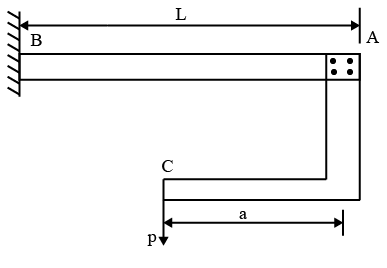# ISRO Scientist or Engineer Mechanical 2010

Instructions

For the following questions answer them individually

Question 21

# A particle is projected at an angle $$\theta$$ to the horizontal and it attains a maximum height H. The time taken by the projectile to reach the highest point, of its path isQuestion 22

# The tension in the cable supporting a lift moving upwards is twice the tension when the lift moves downwards. Whatis the acceleration of the lift?Question 23

# For the apparent weight of a body at equator to become zero, the earth should rotate with an angular velocity ofQuestion 24

# A cantilever beam AB of length L and uniform flexural rigidity EI has a bracket AC attached to its free end shown in Fig. Vertical load is applied to the free end C of the bracket. In order that the deflection of point A to be zero the ratio a/L should beQuestion 25

# Given that$$P_E$$ = the crippling load given by Euler$$P_C$$ = the loadat failure due to direct compression$$P_R$$ = the load in accordance with the Rankine’s criterion of failureThen $$P_R$$ is given byQuestion 26

# Bending moment M and torque is applied on a solid circular shaft. If the maximum bending stress equals to maximum shear stress developed, them M is equal toQuestion 27

# For solid shaft subjected to a torque of 18000 Nm having a permissible shear stress of $$60N/mm^2$$, the diameter of shaft isQuestion 28

# Shear force isQuestion 29

# Maximum deflection in cantilever due to pure bending moment M atits end isQuestion 30

# Maximum shear stress in Mohr’s circle is equal toOR